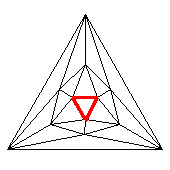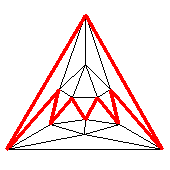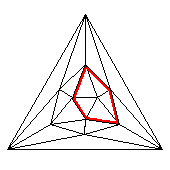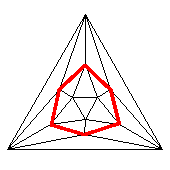### Skew polyhedra

Usually polygons are considered partially ordered sets, having vertices, edges (sides), and some spanned membran (their area or body). In the early 20th century Coxeter and Petrie came up with the concept of skeletal polygons. There only the vertices and edges remain to be considered, whereas the existance of a spanned (flat) membran is no longer required. This then also allows for non-flat and still regular "polygons" like finite or infinite zig-zags, for infinite helices (with finite flat polygonal projection image), or simply the linear apeirogon.

The best-known skeletal polygons however are the Petrie polygons. Those are defined to be skeletal polygons contained within regular polytopes as pseudo faces, which outlines in d-dimensional subelements a sequence of d+1 consecutive edges and then continues accordingly with the neighbouring d-dimensional subelement, where it already had outlined d consecutive edges. As induction start one clearly can take a flat regular polygon. Here the polygon itself and its Petrie polygon coincides. With respect to polyhedra e.g. the Petrie polygons thus are nothing but the equatorial antiprismatic zig-zags. In fact, these Petrie polygons quite generally are eqatorial non-flat zig-zags, allowing for some projection onto a 2-dimensional subspace. Thereby the polytope then will be contained completely within the projection of that zig-zag, which by itself then becomes a flat convex regular polygon.

E.g. within regular polytopes xPoQo...oRo = {P,Q,...,R} the length S of the respective Petrie polygon sometimes gets emphasized additionally by an according index, i.e. {P,Q,...,R}S. It should be noted here however that the latter symbol on the other hand is also being re-used for according mod-wraps, i.e. polytopes, which have the same local structure as {P,Q,...,R}, but globally get narrowed down to a fraction S' of the former length S by means of according identifications, thus yielding {P,Q,...,R}S', cf. e.g. the elliptical polytopes. (Further such mod-wraps e.g. are considered under the name of regular maps.)

It still took some while until such skeletal polygons where considered to be allowable for polytopal faces too. Rather it is a current topic of interest. The earliest according acception maybe was within the definition of the Petrie dual polyhedra. Those are defined to use the same edge skeleton then the starting regular polyhedron, but replaces the former faces by the respective Petrie polygons. This operation then happens to be involutoric, i.e. twice its application returns to the original polyhedron again. In fact there you simply have {P,Q}Rπ = {R,Q}P, which clearly shows this involutoric behaviour.

But much earlier already such skeletal polygons became acceptable for use within vertex figures. This then became the origin for the skew polytopes. Here all the face polygons still remain flat. One further restricts again for local finiteness, esp. dense vertex figures would not be allowed and therefore infinit skeletal polygons are excluded usually as vertex figures.

Besides the Petrie polygons allone also other polygonal skeletal structures can be considered too. These are (here given wrt. a polyhedral surface tiling):

face
closed or unbounded sequence of edges (sides), making at every vertex the sharpest possible bend, being done into the same direction always
Petrie polygon
closed or unbounded sequence of edges (sides), making at every vertex the sharpest possible bend, being done alternatingly in both directions
hole
closed or unbounded sequence of edges (sides), making at every vertex the 2nd sharpest possible bend, being done into the same direction always
2nd order Petrie polygon
closed or unbounded sequence of edges (sides), making at every vertex the 2nd sharpest possible bend, being done alternatingly in both directions
3rd order hole
closed or unbounded sequence of edges (sides), making at every vertex the 3rd sharpest possible bend, being done into the same direction always

etc.©
Icosahedral graph with emphasized face, Petrie polygon, hole, and 2nd-order Petrie polygon repectively. – Higher ordered ones do not make sense for just 5 faces per vertex.

#### Regular skew Petrie-Coxeter polyhedra

Petrie and Coxeter quite early found 3 regular skew polyhedra, all having flat regular convex faces and finite regular skew polygonal vertex figures. Those all happen to be apeirohedra (infinite count of faces) and show up holes (within common sense), providing higher genus patterns. Coxeter writes {P,Q|R} for such a structure. Here P is the (flat) polygonal type of the faces, Q the (skew) polygonal type of the vertex figures, and R the (flat) polygonal type of the tunnel cross section (hole).

Obviously {P,Q|R} = xPoQo|R and (flat) {P,Q} = xPoQo have the same incidence structure. But the former has some further symmetry applied, which circles around the tunnels (holes). In fact, xPoQo|R happens to be a modwrap of xPoQo, which occurs by identification of each R-th vertex on each hole (in the above defined sense) of xPoQo. At least, as far as such a combination of numbers is realizable. Coxeter provides as according requirement (for a representation within 3-dimensional Euclidean space):  2 sin(π/P) sin(π/Q) = cos(π/R).

The skewness and the regularity of the vertex figure (antiprismatic zigzag) implies that here Q has to be even generally. As xPoQo|R and xQoPo|R generally come in dual pairs, the same holds true for P.

Generally it happens that such skew representations of infinite regular polyhedra with flat regular polygons not only occur as embeddings within 3-dimensional Euclidean space, but well also within spherical or hyperbolical space of same local dimensionality. In fact an according mod-wrap x(2P)o(2Q)o|R generally occurs as a facial substructure of xPxRoQoR*a. And the above mentioned appearance in dual pairings then reflects itself just in the interchange of the node symbol types. – The above ones e.g. become
• {4,6|4} as the squares of x2x4o3o4*a = x4o3o4x (chon)
• {6,4|4} as the hexagons of x3x4o2o4*a = o4x3x4o (batch)
• {6,6|3} as the hexagons of x3x3o3o3*a (batatoh)

The finite examples in contrast are to be found as realizations within spherical space. Thus we have
• {4,6|3} as the 30 squares of x2x3o3o3*a = x3o3o3x (spid)
• {6,4|3} as the 20 hexagons of x3x3o2o3*a = o3x3x3o (deca)
• {4,8|3} as the 288 squares of x2x3o4o3*a = x3o4o3x (spic)
• {8,4|3} as the 144 octagons of x4x3o2o3*a = o3x4x3o (cont)
Their incidence matrices then are (resp. their dual ones):

```20 |  6 |  6		144 |   8 |   8
---+----+---		----+-----+----
2 | 60 |  2		  2 | 576 |   2
---+----+---		----+-----+----
4 |  4 | 30		  4 |   4 | 288
```

The easiest spherical space examples however obviously are all these {4,4|R}, which then just describe the R2 torical squares (i.e. lacing squares) of the R-prismatic cells of the general uniform (R,R)-duoprism. Moreover that example also is nothing but the regular map {4,4}(R,0). Their selfdual incidence matrices here clearly are

```RR |   4 |  4
---+-----+---
2 | 2RR |  2
---+-----+---
4 |   4 | RR
```

#### Wythoffian skew polyhedra with flat faces

A direct application of Wythoff's construction advice might not be too obvious here. But the according local operations in the sense of Stott expansions surely apply. Because of the existance of skew vertex figures however, this generally would produce not infinite polyhedra with flat faces only. But a single generally applicable exception each could be listed here, none the less. It is the modwrap of x(2P)o(2Q)x, when identifying each 2R-th vertex on each hole. That one then likewise occurs as according facial substructure of xPxRxQxR*a. In fact there it omits, beside all cell bodies for sure, all 2R-gonal faces.

#### Uniform skew polyhedra with flat faces

Other infinite polyhedra with flat faces and a single skew vertex figure type only, even when restricted to 3-dimensional eucidean embedding space, so far are still not being classified. Many of them simply are obtained as mirror periodic packings of one ore two uniform polyhedra.